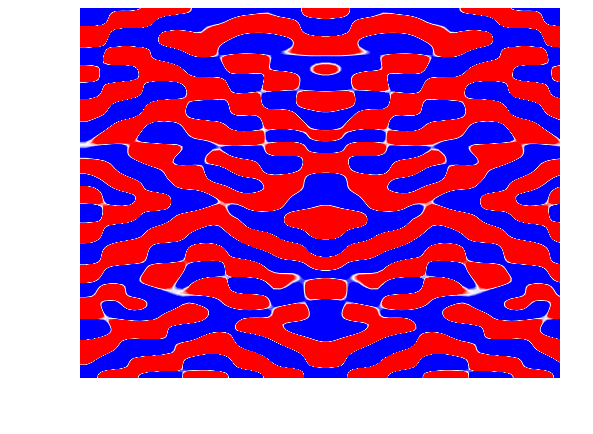Nonlinear-resonance generated low-frequency flow

Relevant publication:

Burns, P., J. Shipton, D. Acreman and B. Wingate. 2020. Frequency interactions of nonlinear resonance in the persistence of density layers as a low-frequency bandwidth (submitted to Geophysical and Astrophysical Fluid Dynamics)

Our work at the University of Exeter (seefor project details) has explored the role of fast waves (i.e. internal waves, which propagate beneath the surface of the fluid) in the creation and longevity of density layers. To explore how fast waves contribute to the persistence of the layers we have combined mathematical theory and numerical simulations. The mathematics tells us that nonlinear resonance, a constructive interaction of internal waves, will cause a low-frequency signal in our system for all time, and our numerical simulations reveal this low-frequency signal to be a layer structure. One focus of our work is exploring the details of the interactions of internal waves that occurs within the nonlinear resonance, to gain a better understanding of exactly how energy is transfered from high frequencies (i.e. the fast waves) to the low-frequency bandwidth. Consider the following equation,

$$\begin{eqnarray} && \frac{\mathrm{d}\overline{\sigma}_{{\boldsymbol k}}^{\alpha}(t)}{\mathrm{d}t}\, +\frac{i}{8}\frac{1}{T} \int_0^T \bigl[ \sum_{\substack{{\boldsymbol k}_1+{\boldsymbol k}_2={\boldsymbol k} \\|n_1-n_2|=n}}\sum_{\alpha_{1},\alpha_{2}} \, C_{ \boldsymbol{k}_1 \boldsymbol{k}_2 \boldsymbol{k}}^{\alpha_1 \alpha_2 \alpha} \, \overline{\sigma}_{{\boldsymbol k}_{1}}^{\alpha_{1}}(t)\,\overline{\sigma}_{{\boldsymbol k}_{2}}^{\alpha_{2}}(t)\, e^{-i\Omega\,s} \bigr] \mathrm{d}s = 0. \end{eqnarray}$$

The above equation is derived from the theory of fast-singular limits, a theory which involves asymptotics, the matrix exponential and wave averaging. The asymptotics, for this problem, includes the assumption of timescale separation, where two physical processes operating on distinct timescales occur simultaneously. In the above equation note the two timescales, one fast ($$s$$) and one slow ($$t$$), and the wave averaging (revealed by the integral over the fast timescale $$s$$, associated with the fast waves). The matrix exponential has the form $$e^{-s\,\mathcal{L}}$$ which results in the exponential terms shown in the equation above. The matrix exponential eliminates the linear term $$\mathcal{L}(\boldsymbol{u})$$ from the original governing equation (not shown), where the linear operator $$\mathcal{L}$$ is the source of fast oscillations in the system. This results in a new equation for the slow dynamics (the equation above). The above equation gives the evolution of the slow dynamics (in spectral space), that is, $$d\overline{\sigma}_{\boldsymbol k}^{\alpha}/dt$$, which is the low-frequency density layers in our system. The theory of fast-singular limits involves taking the limit $$T\to\infty$$ and so considers the slow dynamics to be only the zero frequency. However, here we take a finite integral to derive an approximate wave-averaged equation, which allows for a bandwidth of low-frequency motions, as observed in our numerical experiments. Diffusion has been neglected for simplicity in the above equation. The frequency interactions of fast waves are given by

$$\begin{equation} \Omega = \omega_{{\boldsymbol k}_1}^{\alpha_1} + \omega_{{\boldsymbol k}_2}^{\alpha_2} - \omega_{\boldsymbol k}^{\alpha} = \left({ \frac{|k_1|}{|{\boldsymbol k}_1|}\alpha_1 + \frac{|k_2|}{|{\boldsymbol k}_2|}\alpha_2 - \frac{|k|}{|{\boldsymbol k}|}\alpha }\right) N, \end{equation}$$

where $$\omega_{{\boldsymbol k}_1}^{\alpha_1}$$ and $$\omega_{{\boldsymbol k}_2}^{\alpha_2}$$ are the frequencies of the interacting linear waves, and $$\omega_{{\boldsymbol k}}^{\alpha}$$ is a mode of the solution. It turns out that there is only a significant contribution to the slow dynamics when $$\Omega$$ is small, which is due to the 'cancellation of oscillations' effect. The above equation is therefore showing how the interactions of fast waves is generating a low-frequency signal in the solution. As explained above, one focus of our work is to explore the details of the frequency interactions in $$\Omega$$.

Here are two simulations of layers in our idealised numerical experiments using the spectral-methods model Dedalus:The movies show a layering of the fluid across the domain using contours of the vertical gradient of density. Near-white regions mean the fluid is well mixed. The left movie shows results from a simulation where internal waves were generated from an initial quasi-random buoyancy perturbation but with no flow and the energy in the system was then allowed to decay under diffusion. In contrast the right movie shows the results from a simulation where the flow field was continuously forced using a Gaussian shaped spectrum of wave frequencies. Both approaches have their advantages. The unforced system is simpler and we used it to help elucidate the effects of nonlinear resonance on the longevity of the layers. The signatures of internal waves are evident in the simulations, giving away their secret. It is amazing and perhaps counter-intuitive that these relatively fast moving ephemeral waves cause the long-lived slow layer structure. The right movie shows that the layers persist through time and do not decay away as they do in the left movie. The Gaussian force continuously creates wave motions which via nonlinear resonance then create the low-frequency signal (the layers). This is akin to what happens in our oceans where a spectrum of internal waves is always present, generated mainly by the tides and surface winds.

Density layers frequently occur in the oceans, with one example being the layers of different density observed in the Arctic Ocean pycnocline, for example, during the Beaufort Gyre Exploration Project. The layers have been observed to span almost the entire Arctic basin, and help to limit the upward flow of heat energy from the deeper ocean, essentially shielding the Arctic ice from this energy. If transported to the surface waters this heat in the deep could melt the entire Arctic ice pack. Under a changing climate, changes to ocean circulation are likely to change the layer structure in the Arctic Ocean, which would change the vertical flow of heat and so affect the rate of ice melt. Therefore these layers potentially have a role to play in climate change.

Although the project has focused on idealised systems and ocean phenomena, the fundamental physics and mathematics applies to other analogous systems, for instance, the atmospheres of giant planets, the hot-magnatised plasmas of fusion physics, as well as Earth's atmosphere and stratified lakes. Indeed the phenomena of nonlinear-resonance-generated low-frequency structures occurs wherever a system supports fast waves and whenever timescale separation exists.